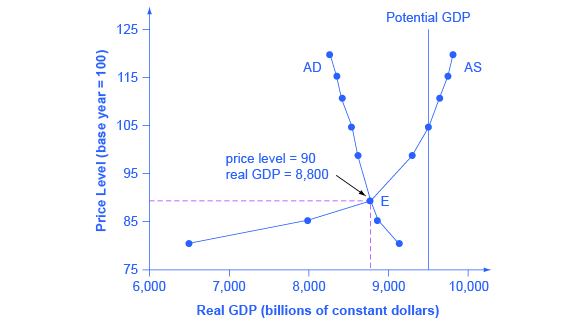# Building a Model of Aggregate Demand and Aggregate Supply

## Equilibrium in the Aggregate Demand/Aggregate Supply Model

The intersection of the aggregate supply and aggregate demand curves shows the equilibrium level of real GDP and the equilibrium price level in the economy. At a relatively low price level for output, firms have little incentive to produce, although consumers would be willing to purchase a large quantity of output. As the price level rises, aggregate supply rises and aggregate demand falls until the equilibrium point is reached.

Figure combines the AS curve from Figure and the AD curve from Figure and places them both on a single diagram. In this example, the equilibrium point occurs at point E, at a price level of 90 and an output level of 8,800.Aggregate Supply and Aggregate Demand The equilibrium, where aggregate supply (AS) equals aggregate demand (AD), occurs at a price level of 90 and an output level of 8,800.

Confusion sometimes arises between the aggregate supply and aggregate demand model and the microeconomic analysis of demand and supply in particular markets for goods, services, labor, and capital. Read the following Clear It Up feature to gain an understanding of whether AS and AD are macro or micro.

## Are AS and AD macro or micro?

These aggregate supply and demand models and the microeconomic analysis of demand and supply in particular markets for goods, services, labor, and capital have a superficial resemblance, but they also have many underlying differences.

For example, the vertical and horizontal axes have distinctly different meanings in macroeconomic and microeconomic diagrams. The vertical axis of a microeconomic demand and supply diagram expresses a price (or wage or rate of return) for an individual good or service. This price is implicitly relative: it is intended to be compared with the prices of other products (for example, the price of pizza relative to the price of fried chicken). In contrast, the vertical axis of an aggregate supply and aggregate demand diagram expresses the level of a price index like the Consumer Price Index or the GDP deflator—combining a wide array of prices from across the economy. The price level is absolute: it is not intended to be compared to any other prices since it is essentially the average price of all products in an economy. The horizontal axis of a microeconomic supply and demand curve measures the quantity of a particular good or service. In contrast, the horizontal axis of the aggregate demand and aggregate supply diagram measures GDP, which is the sum of all the final goods and services produced in the economy, not the quantity in a specific market.

In addition, the economic reasons for the shapes of the curves in the macroeconomic model are different from the reasons behind the shapes of the curves in microeconomic models. Demand curves for individual goods or services slope down primarily because of the existence of substitute goods, not the wealth effects, interest rate, and foreign price effects associated with aggregate demand curves. The slopes of individual supply and demand curves can have a variety of different slopes, depending on the extent to which quantity demanded and quantity supplied react to price in that specific market, but the slopes of the AS and AD curves are much the same in every diagram (although as we shall see in later chapters, short-run and long-run perspectives will emphasize different parts of the AS curve).

In short, just because the AD/AS diagram has two lines that cross, do not assume that it is the same as every other diagram where two lines cross. The intuitions and meanings of the macro and micro diagrams are only distant cousins from different branches of the economics family tree.